# Quantitative Aptitude Quiz for IBPS 2020 Mains Exams- 20th December

Directions (1-5): Table given below shows total number of votes cast in five cities. Total votes cast to X (in percentage) and total invalid votes cast. Only two people participate in the election (i.e., X and Y). Study the data carefully & answer the following questions.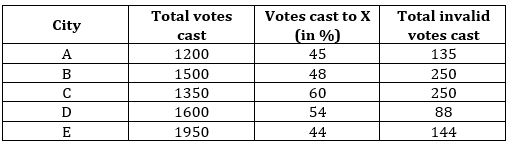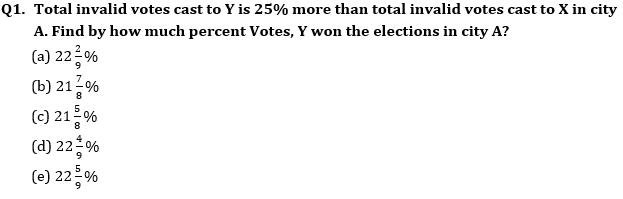Q2. Find the average number of total votes cast to Y in city A, C and E together?
(a) 736
(b) 748
(c) 764
(d) 754
(e) 760

Q3. Total invalid votes cast to Y in city D and city E is 25% and 50% respectively. Then total valid votes cast to Y in city D is what percent of total valid votes cast to Y in city E.
(a) 80%
(b) 75%
(c) 65%
(d) 60%
(e) 70%

Q4. Total votes cast to X in city C and D together is what percent of the total votes cast to Y in city A and B together?
(a) 110.25%
(b) 112.75%
(c) 114.25%
(d) 116.25%
(e) 118.25%

Q5. Total valid votes cast to Y in city B is how much more than total valid votes cast to Y in city C if total invalid votes cast to X in city B and City C is 84% and 92% respectively.
(a) 210
(b) 220
(c) 230
(d) 240
(e) 250

Directions (6-10): What approximate value should come in place of question mark (?)in the following questions?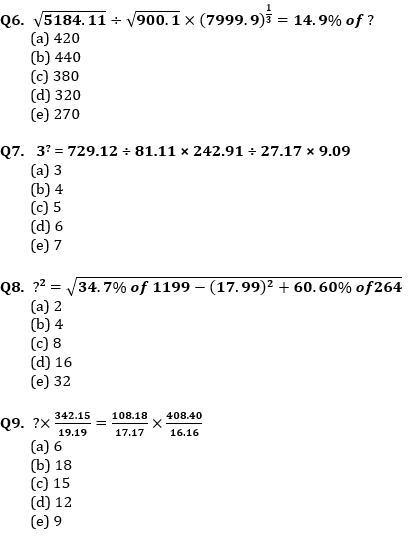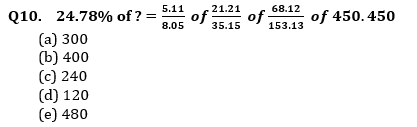Directions (11-15): Pie-chart given below shows population of two cities travel by five modes of transportation. Study the chart carefully and answer the following question.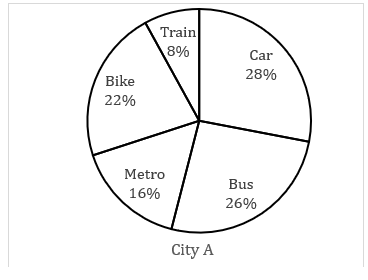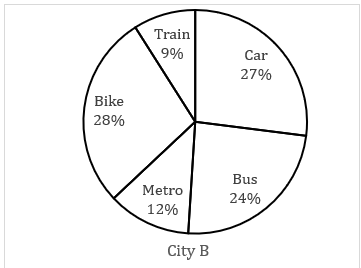Note:- Ratio of total population of city A to city B is 5 : 2.

Q11. Total number of person travel by bike in city A is 945 more than that of in city B, then find the total number of person who travel by bus in city A and city B together?
(a) 3010
(b) 3115
(c) 3055
(d) 3085
(e) 3145

Q12. Number of females travel by car in city B is 25% more than number of males travel by car in city B, which is 25% of number of males travel by car in city A. If total number of person travel by metro in city B is 456, then find the number of females travel by car in city A.
(a) 570
(b) 1140
(c) 1824
(d) 836
(e) 912

Q13. If total number of person travel by car from city A and city B together is 582, then total number of person travel by bike and metro together from city A is what percent more than total number of person travel by bike and metro together from city B?
(a) 37.5%
(b) 50%
(c) 137.5%
(d) 150%
(e) 237.5%

Q14. If number of person travel by car in city B is 126 more than number of person travel by train in city A, then find the average number of person travel by metro in city A and B together?
(a) 468
(b) 364
(c) 414
(d) 428
(e) 442

Q15. Total number of person in city B who travel by metro and train together is how much more than total number of person in city A who travel by train. If it is given that total population of city B is 95 more than total number of person in city A who travel by bike and metro together.
(a) 13
(b) 15
(c) 17
(d) 19
(e) 21

Practice More Questions of Quantitative Aptitude for Competitive Exams:

###### Mains Quiz Study Plan for IBPS Exams 2020

Solutions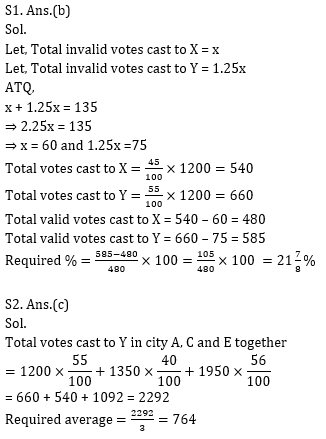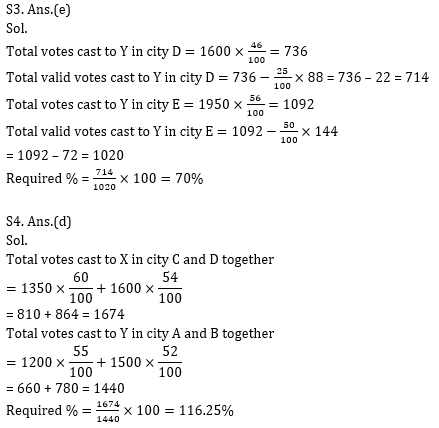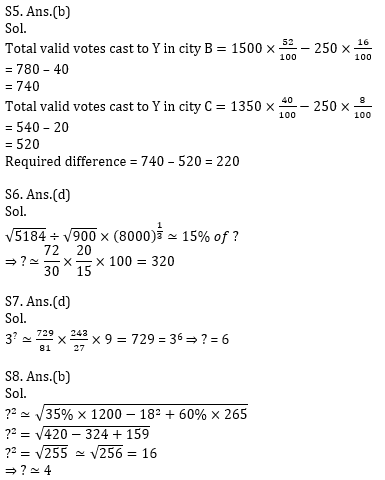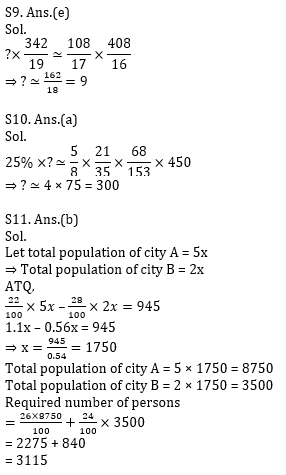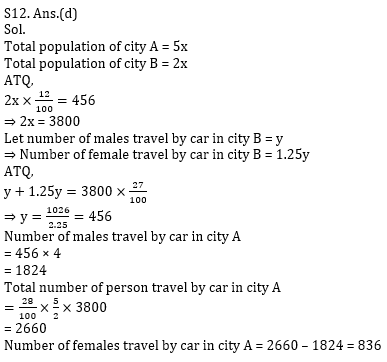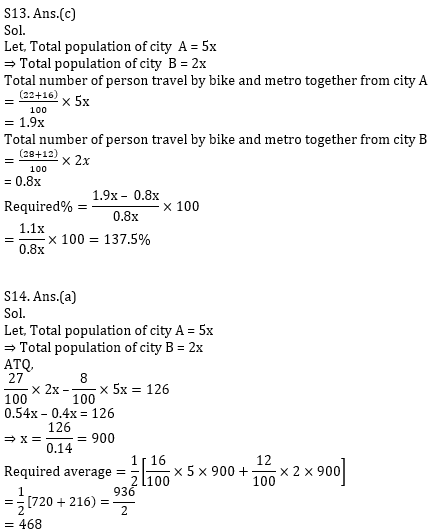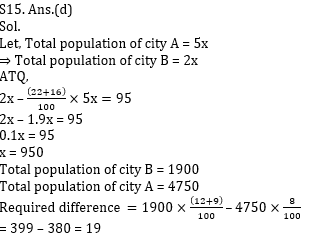Practice with Crash Course and Online Test Series for IBPS Clerk Prelims: